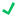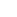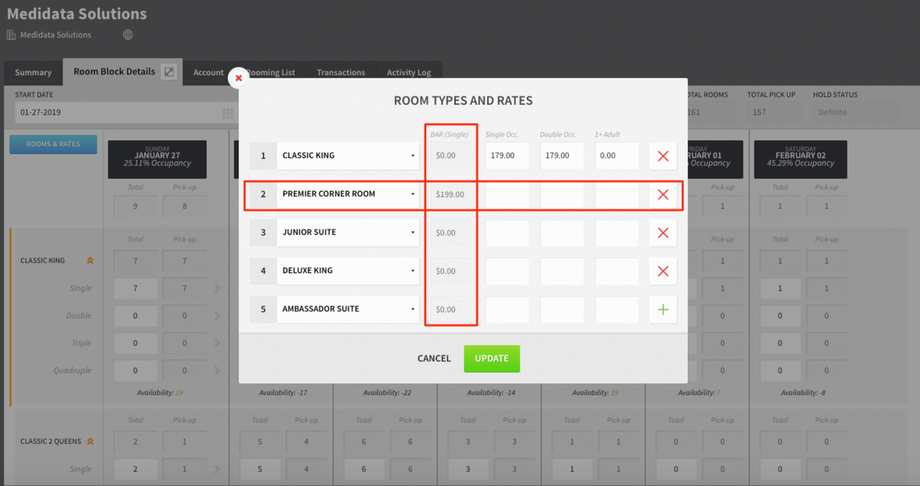# How the BAR (Single) Column Is Populated

Modified on: Mon, 16 Sep, 2019 at 3:52 PM

Applies to:Rover PMSRover Overlay

# How the BAR (Single) Column Is Populated

On the room block details tab of a group, when you look at room and rates, there is a column called BAR (for Best Available Rate). Below is the logic of how this is populated:

1. Consider a room type RT.
2. Rate A, Rate B, and Rate C are the rates in the hotel.
3. The single amounts for Rate A, Rate B, and Rate C, are \$10, \$15, and \$5, respectively.
4. Here, the room type RT is only configured for Rate A and Rate C.
5. So, a BAR of RT = MINIMUM (single amount [Rate A, Rate C]) = MINIMUM (\$10, \$5) = \$5.

BAR is the minimum single amount of the rates (single amount is set in Rate Manager for the group FROM date) in which the room type is configured. We will not consider the double amount, extra adult amount, and child amount for groups.Did you find it helpful? Yes No

Send feedback